What is Voltage and Current?

## ?

Key Terms

o         Coulomb

o         Voltage

o         Potential energy

o         Kinetic energy

o         Volt

o         Current

o         Ampere (amp)

Objectives

o         Learn how to quantify electric charge

o         Define voltage and current in relation to electric charge

You've probably heard of voltage, current, and power in the context of electricity, but you may or may not be aware of their precise meanings. Common parlance about electricity also tends to obscure these concepts. Therefore, this article will provide you a scientifically correct understanding of the meaning of these critical parameters, facilitating our later discussion of electronic circuits and devices.

Quantifying Charge

Since the electric force is a result of the interaction of charge, we must first be able to quantify charge before we can accurately discuss related quantities like current and voltage. As we discussed earlier, electrons and protons--two subatomic particles found in atoms--are charged: that is, they have a certain quality that gives rise to the electric force. As it turns out, all electrons (protons) have the same amount of negative (positive) charge. An electron and proton carry the same magnitude (or "amount") of charge, but the electron's charge is defined as negative and the proton's charge as positive. (Note that this is an arbitrary definition--the main point is that the two types of charges are opposite.) Let's call the magnitude of charge in a proton e.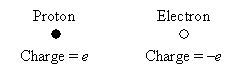The SI (International System of Units) unit for charge is the coulomb, which is defined as an amount of charge equivalent to that of about 6,250,000,000,000,000,000 protons (expressed in scientific notation as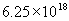)--a huge number, but it's not really that much matter when you consider how tiny a proton is! Of course, even defined this way, a coulomb may not seem that meaningful to you: let's just say it's an arbitrary amount of charge that we define as our unit (much like we might arbitrarily define a standard of length, such as a foot or meter).

Interested in learning more? Why not take an online Electronics course?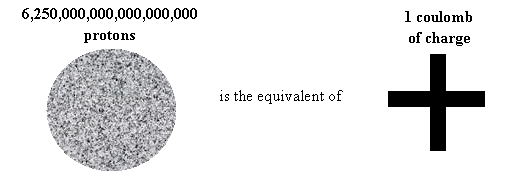In the same way, 6,250,000,000,000,000,000 electrons is the equivalent of negative one coulomb of charge.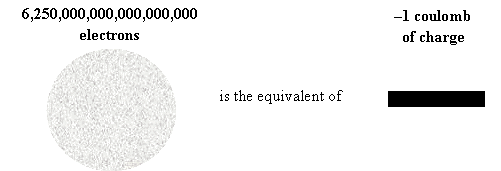Remember, a coulomb is just an arbitrarily defined amount that we'll use as a "yard stick" to measure charge.

Voltage

If you look at a battery, you'll notice that (in addition to its size--AA, D, C, and so on) it is specified by its voltage: 1.5 volts, for example. Other devices specify the voltages they require to operate. Typical wall sockets (in America) provide about 120 volts. But what exactly is voltage? Voltage is a measure of potential energy --the amount of energy "stored" in an object. Let's try to understand this by illustrating potential energy using a more familiar context: gravity.

Consider the floor of your room to be "ground level," and set an object (such as a ball) on the floor. Relative to ground level, that ball has no gravitational potential energy, because when you release it, it doesn't accelerate. Now, hold the ball some distance above the floor. The ball now has a certain potential energy because when you release it, it accelerates until it hits the floor (at which point all its potential energy has been converted by gravity into kinetic energy --energy of motion).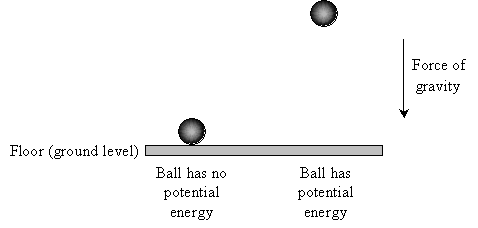And as you probably know, the higher off the ground you hold the ball, the faster it will be traveling when it finally reaches the floor once you release it (ignoring air resistance).

Voltage is very similar. Instead of masses (such as a ball) experiencing gravity, however, we are dealing with charged objects that feel an electric force. Let's say our ball has 1 coulomb (1C) of charge and that it is experiencing an electric force that is directed downward (similar to gravity). We'll pick some point and call it "ground level" (or just "ground"). Then, the ball has no (electric) potential energy when it is at ground level, but if it is moved away from ground level, it has (electric) potential energy--just as with gravity.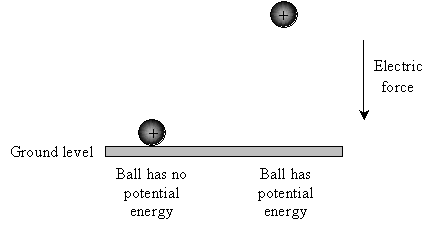But where might such a force come from? Recall that charges attract or repel one another. Let's say we have a metal plate infused with excess electrons (giving it an overall negative charge). Place the negatively charged plate at "ground level." Because positive and negative charges attract one another, an electric force is created between the ball and the plate; when released, the ball held away from the plate will accelerate toward the plate, but the ball in contact with the plate will remain motionless.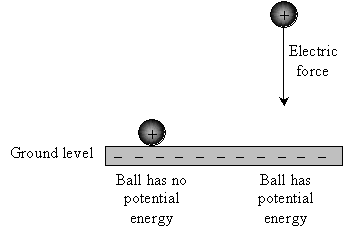Voltage is a measure of this potential energy. Specifically, voltage is the amount of potential energy an object has at a given place--relative to some pre-defined "ground level"--per coulomb of charge in that object. So, to return to the illustration above, if the plate is infused with more negative charge, the voltage at a specific point above the plate will increase. Likewise, if less negative charge is present in the plate, the voltage at that same point will decrease.

Because voltage is defined by a ground level and some point away from ground, voltage is always and only a measurement between or across two points. For example, in the case of a battery, the voltage is a measure of potential energy between one terminal (end) of the battery and the other. In other words, a voltage at any point is always relative to some pre-defined ground level.

The SI unit for voltage is (not surprisingly) the volt, which is defined as a potential energy of one joule per coulomb of charge (a joule, like a coulomb, is just an arbitrarily defined unit of energy). For our purposes, just remember that a volt is just a measure of how much potential energy a charged object has at a particular location relative to ground.

Current

Another important unit is current, which is much easier to understand than voltage. Let's illustrate current using the example of a wire (which is nothing more than a thin conductor). Let's also say there's a potential energy difference (that is, a voltage) between the two ends of the wire, which causes positive charges to move from one end to the other. We'll define one end of the wire as "ground level" (or simply "ground").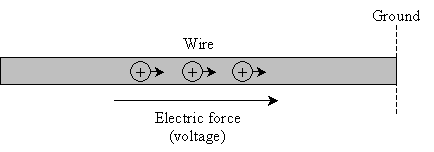Current is nothing more than the amount of charge that passes through the wire. In particular, we define current at a specific point: the current is the number of coulombs of charge passing by that point per second.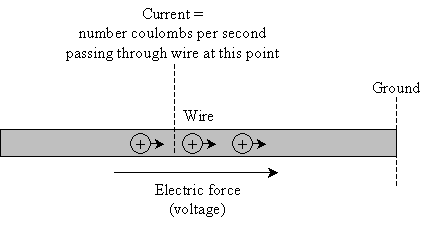In many cases, a stronger electric force (meaning a higher voltage) produces a higher current, because the charges are pulled faster toward ground.

The SI unit for current is the ampere (sometimes just called an amp ), which is defined as a flow of 1 coulomb per second, also written as 1A.

Practice Problem: A simple electrical circuit is shown below, with voltages identified at various points. At which of these points would a coulomb of charge have the most potential energy?

 Electron (negative charge)Solution: Voltage is a measure of potential energy. A coulomb of charge placed at point A would have 12 joules of potential energy; if it is placed at point B, it has 3 joules of potential energy. In an "electrical sense," these points are different distances from ground, leading to different electric potential energies, just as a ball held at different heights has different gravitational potential energies. For the simple circuit diagram shown above, a coulomb of charge would have the most potential energy at point A.

Practice Problem: A wire in an electrical circuit is conducting 3A (3 amps). How many coulombs of charge pass any given point in the wire each second?

Solution: As we discussed above, an ampere (amp) is a current equivalent to 1 coulomb per second. Thus, 3 amps is the same as 3 coulombs per second. Thus, at any point in a wire conducting 3A, 3 coulombs are passing every second.